Reach Us+44-7482-875032
Peristaltic Pumping of Johnson-Segalman Fluid in an Asymmetric Channel under the Effect of Hall and Ion-Slip Currents | OMICS International
Journal of Applied & Computational Mathematics
All submissions of the EM system will be redirected to Online Manuscript Submission System. Authors are requested to submit articles directly to Online Manuscript Submission System of respective journal.

# Peristaltic Pumping of Johnson-Segalman Fluid in an Asymmetric Channel under the Effect of Hall and Ion-Slip Currents

Kh. Nowar1*, E. Abo-Eldahab2 and E. Barakat2

1Department of Mathematics, Faculty of Science, King Saud University, Riyadh, Saudia Arabia

2Department of Mathematics, Faculty of Science, Helwan University, Helwan, Egypt

*Corresponding Author:
Kh. Nowar
Department of Mathematics
Faculty of Science, King Saud University|
Riyadh, Saudia Arabia
E-mail: [email protected]

Received March 03, 2012; Accepted April 08, 2012; Published April 12, 2012

Citation:Nowar Kh, Abo-Eldahab E, Barakat E (2012) Peristaltic Pumping of Johnson-Segalman Fluid in an Asymmetric Channel under the Effect of Hall and Ion-Slip Currents. J Applied Computat Mathemat 1:102. doi: 10.4172/2168-9679.1000102

Copyright: © 2012 Nowar Kh, et al. This is an open-access article distributed under the terms of the Creative Commons Attribution License, which permits unrestricted use, distribution, and reproduction in any medium, provided the original author and source are credited.

Visit for more related articles at Journal of Applied & Computational Mathematics

#### Keywords

Johnson-Segalman fluid; Hall currents; Ion-slip; Asymmetric channel; Peristalsis

#### Introduction

Peristaltic pumping is a special kind of transport, in which, physiological fluids may be pumped from one place in the body to another place. Peristaltic motion flows attracted the attention of many researchers because it is widely observed in industry and biology. Intense research on peristalsis has been done and is still demanded because of its useful applications. Such applications include enhanced oil recovery, chemical processes such as in distillation towers and fixed-bed reactors, urine transport from kidney to bladder through the ureter, transport of lymph in the lymphatic vessels, swallowing food through the esophagus, the movement of chyme in the gastrointestinal tract, ovum movement in the fallopian tube, transportation of spermatozoa in the ductus efferentes of the male reproductive tracts, in the vasomotion of small blood vessels, in sanitary fluid transport, and blood pumps in heart lung machine. In addition, peristaltic pumping occurs in many practical applications involving bio-mechanical systems. The peristaltic flows can be divided to Newtonian and non-Newtonian flows that have been reported analytically, numerically, and experimentally by a number of researchers [1-11]. Although most prior studies of peristaltic transport have focused on Newtonian fluids, there are also studies involving non- Newtonian fluids [12-20] i.e. fluids in which the relation between shear stress and shear rate is not linear.

The Johnson-Segalman fluid is one of the developed models to predict non-Newtonian effects. This model is a viscoelastic fluid model which was developed to allow for non-affine deformations . Some investigations [22-24] have studied this model to explain the phenomenon of spurt. The term spurt is used to describe the large increase in the volume throughout for a small increase in the driving pressure gradient at a critical pressure gradient. Hayat et al.  examined the peristaltic flow of a magnetohydrodynamic Johnson- Segalmann fluid for planner channel and found the perturbation solutions and general solutions using symmetry method. Elshahed and Haroun  studied the peristaltic transport of Johnson-Segalman fluid under the effect of magnetic field. Haroun  considered the peristaltic flow of an inclined asymmetric channel for fourth grade fluid. Srinivas and Pushparaj  discussed the non-linear peristaltic transport in an inclined asymmetric channel. Hayat et al.  investigated the peristaltic transport of a Johnson-Segalman fluid in an asymmetric channel. Reddy and Raju  studied the non-linear peristaltic pumping of Johnson-Segalman fluid in an asymmetric channel under the effect of a magnetic field. Abo-Eldahab et al. [30-31] investigated the effects of Hall and ion-slip currents on magnetohydrodynamic peristaltic transport and couple stress fluid.

The aim of this paper is to investigate the effect of Hall and ionslip currents on peristaltic transport of a Johnson-Segalman fluid in an asymmetric channel. We introduce the governing equations and boundary conditions as mentioned below. The perturbation solution for small Weissenberg number is mentioned below. Numerical results, discussions and conclusions are also mentioned below.

#### Problem Formulation

Consider the peristaltic flow of an incompressible Johnson- Segalman fluid in a two dimensional infinite asymmetric channel of width d1+d2. The asymmetry in the channel is produced by assuming the peristaltic wave train on the channel walls traveling with the same speed c but with different amplitudes and phases as shown in Figure 1; The walls of the channel are defined as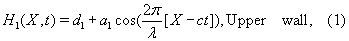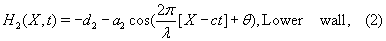Where a1, a2 are the amplitudes of the upper and lower waves, λ is the wave length, θ is the phase difference and t is the time. Note that θ = 0 corresponds to an asymmetric channel with waves out of phase and θ = π describes the case where waves are in phase. Further, d1, d2, a1, a2, and θ satisfy the following inequality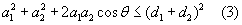so that the walls will not intersect with each other.

The fundamental equations governing the flow of an incompressible Johnson-Segalman fluid together with the generalized Ohm’s law taking the effects of Hall and ion-slip currents, and Maxwell’s equations into account are: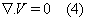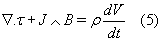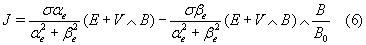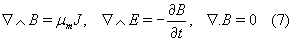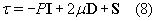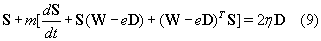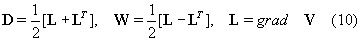where,

V is the velocity vector, ρ is the density of the fluid,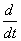is the material derivative, τ is the Cauchy stress tensor, J is the current density, Bis the total magnetic field, E is the total electric field, σ is the electric conductivity, μm is the electric permeability, and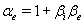βi is the ion-slip parameter, and βe is the Hall parameter. Further, P is the pressure, μ and η are the dynamic viscosities, m is the relaxation time, e is the slip parameter and D, W are the symmetric and skew symmetric part of the velocity gradient, respectively. It should be noted that this model includes the viscous Navier-Stokes fluid as a special case for m= 0. Further, when e = 1 the Johnson-Segalman model reduces to the Oldroyd-B fluid; and when μ = 0 and e = 1, the model reduces to the Maxwell fluid. It is assumed that there is no applied or polarization voltage so that E = 0. Now we assume that a magnetic field B = (0, 0, B0) with a constant magnetic flux density B0 is applied orthogonal to the channel. Neglecting the induced magnetic field under the assumption that the magnetic Reynolds number is small, we get from (6) that the magnetohydrodynamic force is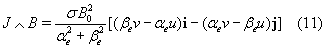Following Shapiro et al.  we introduce a wave frame of reference (x, y) moving with velocity c in which the motion becomes independent of time when the channel is an integral multiple of the wavelength and the pressure difference at the ends of the channel is a constant. The transformation from the fixed frame of reference (X, Y) to the wave frame of reference (x, y) is given by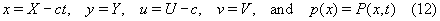Where (u, v), p and (U, V), P are the velocity components and pressure in the wave and fixed frames of reference respectively.

The governing equations in a wave frame of reference are given by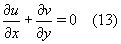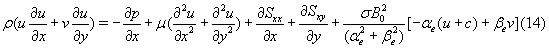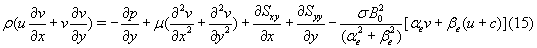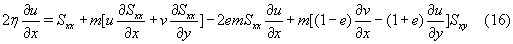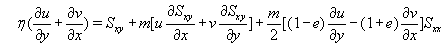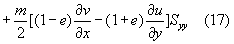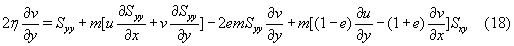Introducing the following non-dimensional variables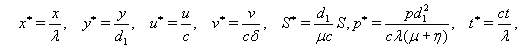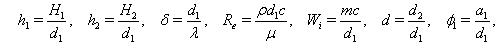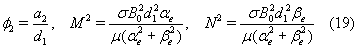into equations (13)-(18) and dropping the stars we get;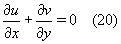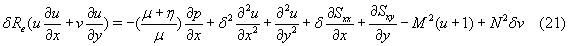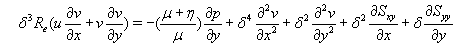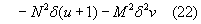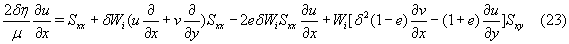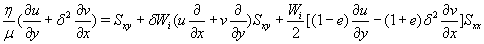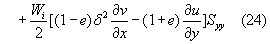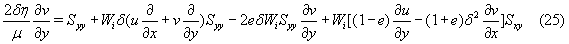Assuming that the wavelength is long and since the Reynolds number is low, then the equations (21)-(25) become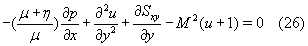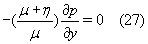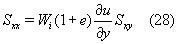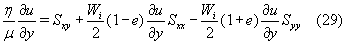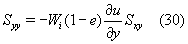Using (28) and (30) in (29) we get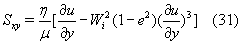From (26) and (31) we can get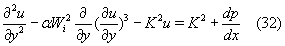where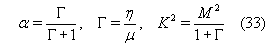The corresponding boundary conditions are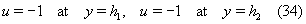where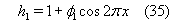and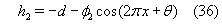#### Rate Of Volume Flow

The volume flow rate in wave frame of reference is given by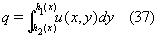where h1, h2 are functions of x alone.

The instantaneous volume flow rate in the fixed frame is given by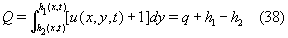in which h1, h2 are functions of x and t.

The time-mean flow over time period T= λ/c is given by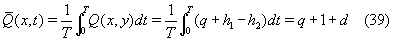Method Of Solution

It is not possible to get an exact solution for equation (32) because, it is a nonlinear differential equation, so we focus our attention to find analytical solution by using the perturbation method. By expanding the flow quantities in a power series of the small parameter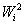as follows: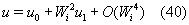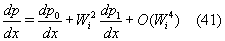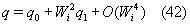Substituting from (40)-(42) into (32) and (34) and collecting terms according to powers of Wi and identifying the coefficients of like powers of Wi we obtain the following systems.

Zeroth-order system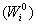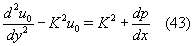The corresponding boundary conditions are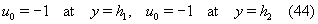First-order system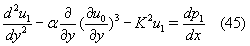The corresponding boundary conditions are

u1 = 0 at y = h1>, u1 = 0 at y = h2 (46)

Zeroth-order solution

The general solution of (43) can be easily obtained in the form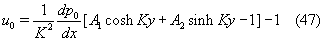By usi ng (44) we find that the constants a1 and a2 take the forms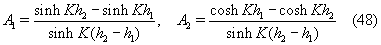The volume flow rate q0 is given by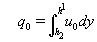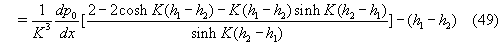From (49) we can write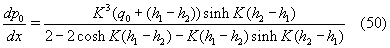First-order solution

Substituting from (47) into (45) and solving (45) we get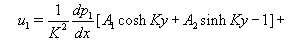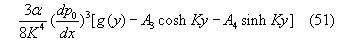where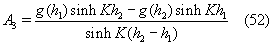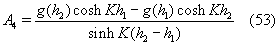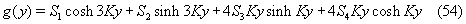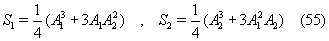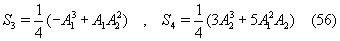the volume flow rate is given by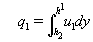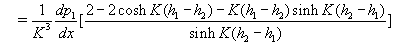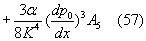and,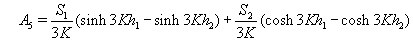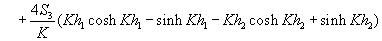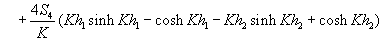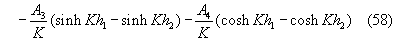From (57) we can obtain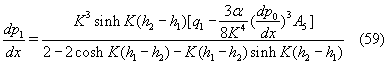substituting from (50) and (59) into (41), taking (42) into account, and neglecting terms of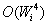we get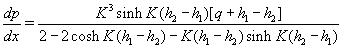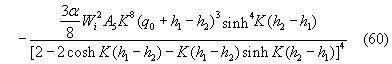The dimensionless pressure rise per one wavelength in the wave frame is defined as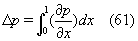#### Results and Discussion

This section represents the graphical results in order to be able to discuss the quantitative effects of the sundry parameters involved in the analysis.

The variation of the axial pressure gradient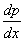with x for various values of Wi, Γ, βe, and βi are shown in Figure 2. Figure (2a) studies the effects of Weissenberg number W1 on the axial pressure gradientand it is noticed that the axial pressure gradient increases by increasing Weissenberg number. Further it is observed that the axial pressure gradient is more for Johnson-Segalman fluid (0 < Wi < 1) than that of Newtonian fluid (Wi = 0). Figure (2b) shows the variation of the axial pressure gradientwith viscosity ratio Γ. It is clear that the axial pressure gradient increases with an increase in viscosity ratio. In Figures (2c) and (2d) it is obvious that the axial pressure gradient has not affected by increasing the Hall and ion-slip parameters.

Figure 3 illustrates the variation of the axial pressure gradientwith x for different values of θ, φ1, φ2 and d. It is clear that the axial pressure gradient decreases by increasing the phase shift θ (Figure (3a)), and also decreases by increasing the width of the channel d (Figure (3d)). The situation is reversed in Figures (3b) and (3c), the axial pressure gradient is increased with an increase in φ1 and φ2.

In Figure 4 we studied the variation of time average flux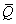with Δp for different values of θ, βe, βi and Γ. Figure (4a) declares that the time average fluxdecreases by increasing the phase shift θ in both co-pumping (Δp < 0) and free pumping (Δp = 0), while it increases by increasing the phase shift θ in the pumping region (Δp > 0). Figures (4b), (4c), (4d) reveal that, the time average fluxdecreases by increasing the Hall parameter βe (Figure (4b)), the ion-slip parameter βi (Figure (4c)), and the viscosity ratio Γ (Figure (4d)) in each of the three regions.

Figure 5 considered the variation of time average fluxwith Δp for different values of φ1 φ2, d, and Wi. It is obvious from Figures (5a), (5b), and (5c) that the time average flux increases by increasing φ1 , φ2, and d in the co-pumping and free pumping regions, also it decreases by increasing them in the pumping region. The variation of time average fluxwith Δp for different values of Weissenberg number Wi studied in Figure (5d), and it is observed that the time average flux decreases by increasing Weissenberg number in each of the three regions.

#### Conclusions

In the present paper, the effects of Hall and ion-slip currents on the peristaltic transport of a Johnson-Segalman fluid in an asymmetric channel under assumptions of a constant external magnetic field, low Reynolds number, and long wavelength are investigated. The governing equations are first modeled and then solved analytically. The effects of various emerging parameters on the axial pressure gradient and time average flux are observed from the graphs. The results are summarized as follows:

The axial pressure gradientincreases by increasing any of Weissenberg number Wi, viscosity ratio Γ, and upper or lower wave amplitudes φ1 or φ2.

The axial pressure gradientis not affected by increasing any of Hall or ion-slip parameters βe and βi

Increasing the phase shift θ or the width of the channel d decreases the axial pressure gradient.

The time average fluxdecreases by increasing any of Hall parameter βe, ion-slip parameter βi, viscosity ratio Γ, and Weissenberg number Wi.

An increase in the phase shift θ decreases the time average fluxin the co-pumping and free pumping regions and increases it in the pumping region.

The time average fluxincreases by increasing any of upper or lower wave amplitude ( φ1 or φ2), and the width of the channel d in the co-pumping and free pumping regions and it decreases by increasing them in the pumping region.

#### References

Select your language of interest to view the total content in your interested language

### Article Usage

• Total views: 4865
• [From(publication date):
May-2012 - Aug 20, 2019]
• Breakdown by view type
• HTML page views : 1046
• PDF downloads : 3819

## Post your commentCan't read the image? click here to refresh
###### Peer Reviewed Journals

Make the best use of Scientific Research and information from our 700 + peer reviewed, Open Access Journals

International Conferences 2019-20

Meet Inspiring Speakers and Experts at our 3000+ Global Annual Meetings

Top